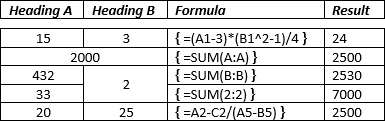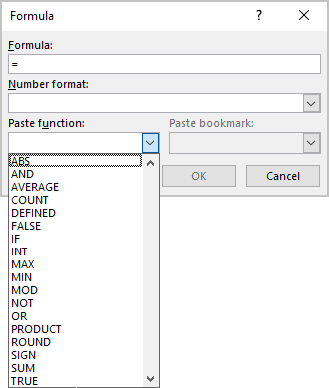# Functions and formulas that you can use in a Word document

Word
Word provides some simple formulas and functions without need to embed Excel tables. It is easy to insert and use formulas:

You can use simple formulas in Microsoft Word, such as addition (+), subtraction (-), multiplication (*), or division (/). Also, you can calculate a power of (^):See How to reference a cell of a Word table for more details.

All functions you can see in the Paste function drop-down list of the Formula dialog box:Function Description
ABS () Calculates the absolute value of the value inside the parentheses.
AND () Evaluates whether the arguments inside the parentheses are all TRUE.
AVERAGE () Calculates the average of the elements identified inside the parentheses.
COUNT () Calculates the number of elements identified inside the parentheses.
DEFINED () Evaluates whether the argument inside parentheses is defined. Returns 1 if the argument has been defined and evaluates without error, 0 if the argument has not been defined or returns an error.
FALSE Always returns 0
IF () Evaluates the first argument. Returns the second argument if the first argument is true; returns the third argument if the first argument is false.

Note: Requires exactly three arguments.

INT () Rounds the value inside the parentheses down to the nearest integer.
MAX () Returns the maximum value of the items identified inside the parentheses.
MIN () Returns the minimum value of the items identified inside the parentheses.
MOD () Takes two arguments (must be numbers or evaluate to numbers). Returns the remainder after the second argument is divided by the first. If the remainder is 0 (zero), returns 0.0.
NOT Evaluates whether the argument is true. Returns 0 if the argument is true, 1 if the argument is false. Mostly used inside an IF formula.
OR () Takes two arguments. If both are false, returns 0, else returns 1. Mostly used inside an IF formula.
PRODUCT () Calculates the product of items identified inside the parentheses.
ROUND () Rounds the first argument to the number of digits specified by the second argument. If the second argument is greater than zero (0), first argument is rounded down to the specified number of digits. If second argument is zero (0), first argument is rounded down to the nearest integer. If second argument is negative, first argument is rounded down to the left of the decimal.
SIGN () Takes one argument that must either be a number or evaluate to a number. Evaluates whether the item identified inside the parentheses if greater than, equal to, or less than zero (0). Returns 1 if greater than zero, 0 if zero, -1 if less than zero.
SUM () Calculates the sum of items identified inside the parentheses.
TRUE Always returns 1.

The arguments can be:

Argument Description
ABOVE Cells above the current. For example, =SUM(ABOVE)
BELOW Cells below the current. For example, =SUM(BELOW)
LEFT Cells on left of the current. For example, =SUM(LEFT)
RIGHT Cells on right of the current. For example, =SUM(RIGHT)